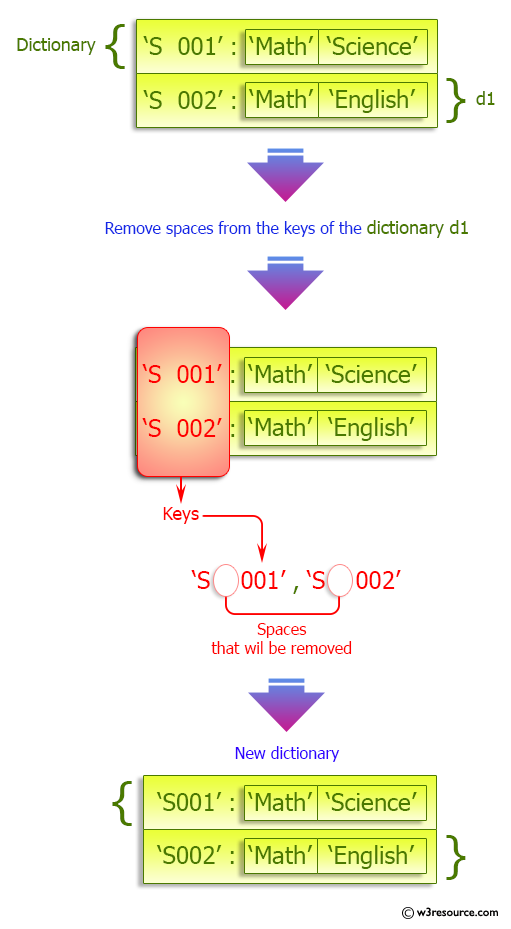﻿ Python: Remove spaces from dictionary keys - w3resource# Python: Remove spaces from dictionary keys

## Python dictionary: Exercise-29 with Solution

Write a Python program to remove spaces from dictionary keys.

Sample Solution:-

Python Code:

``````student_list = {'S  001': ['Math', 'Science'], 'S    002': ['Math', 'English']}
print("Original dictionary: ",student_list)
student_dict = {x.translate({32: None}): y for x, y in student_list.items()}
print("New dictionary: ",student_dict)
```
```

Sample Output:

```Original dictionary:  {'S  001': ['Math', 'Science'], 'S    002': ['Math', 'English']}
New dictionary:  {'S001': ['Math', 'Science'], 'S002': ['Math', 'English']}
```

Pictorial Presentation:<

## Visualize Python code execution:

The following tool visualize what the computer is doing step-by-step as it executes the said program:

Python Code Editor:

Have another way to solve this solution? Contribute your code (and comments) through Disqus.

What is the difficulty level of this exercise?

Test your Programming skills with w3resource's quiz.

﻿

## Python: Tips of the Day

Clamps num within the inclusive range specified by the boundary values x and y:

Example:

```def tips_clamp_num(num,x,y):
return max(min(num, max(x, y)), min(x, y))
print(tips_clamp_num(2, 4, 6))
print(tips_clamp_num(1, -1, -6))
```

Output:

```4
-1
```